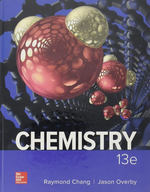### Solution Found!# Intermolecular Forces in HCl, F?, and C?H??

Chapter 11, Problem 11.2.1

(choose chapter or problem)

Get Unlimited Answers! Check out our subscriptions
QUESTION:

What intermolecular forces exist between molecules of the following substances: (a) $$\rm{HCl}$$, (b) $$\mathrm{F}_{2}$$, (c) $$\mathrm{C}_{6} \mathrm{H}_{14}$$?

##### Intermolecular Forces in HCl, F?, and C?H??Want To Learn More? To watch the entire video and ALL of the videos in the series:

Explore the fascinating world of intermolecular forces in this brief video, featuring HCl, F?, and C?H?? molecules. Learn about the bonds that hold these substances together!

QUESTION:

What intermolecular forces exist between molecules of the following substances: (a) $$\rm{HCl}$$, (b) $$\mathrm{F}_{2}$$, (c) $$\mathrm{C}_{6} \mathrm{H}_{14}$$?

Step 1 of 4

The attraction force present between two molecules to hold them tight together is known as an intermolecular force which is of the following types:

a. London dispersion force

b. Dipole-dipole interaction

c. Ion-Ion Interaction

d. Hydrogen bonding